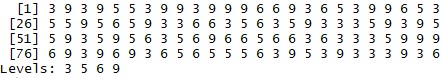# How to Convert a Factor to Numeric in R

Basic R Syntax:

`x_num <- as.numeric(as.character(x))`

More explanations needed? You’ll find all relevant information for the conversion of R factors to numeric in the following tutorial.

## Example: Convert Factor to Numeric in R

To convert a factor to numeric in R can be a tricky task. In the following, I’m therefore going to explain how to convert a factor vector to numeric properly without a loss of information.

Before we can start, we need to create an example factor vector in R:

```set.seed(13579)                                            # Set seed
x <- as.factor(sample(c(3, 5, 6, 9), 100, replace = TRUE)) # Example factor vector```

Our factor vector consists of the four categories 3, 5, 6 & 9:

`x                                                          # Print x to RStudio console`Graphic 1: Example Factor Vector Printed to the RStudio Console

So, how can we transform this factor vector to numeric?

```x_num <- as.numeric(as.character(x))                       # Convert factor to numeric
x_num                                                      # Print converted x to RStudio console
# 3 9 3 9 5 5 3 9 9 3 9 9 9...```

That’s it! Easy, right?

However, please note that – before the conversion to numeric – it is important to transform the factor to character first (as shown in the example above).

I’ll show you why this preliminary step is needed:

```x_wrong <- as.numeric(x)                                   # Wrong conversion to numeric
x_wrong                                                    # Print wrongly converted x to RStudio console
# 1 4 1 4 2 2 1 4 4 1 4 4 4...```

1? 4?! 22??? What happened?! Why does the converted vector consist of wrong values?

The answer is simple: R automatically assigns the numbers 1, 2, 3, 4, and so on to the categories of our factor. If we want to hinder R from doing so, we need to convert the factor to character first.

Keep this in mind, when you convert a factor vector to numeric! I’ve seen this mistake quite often in the past.

## Programming Video: Further Examples

Check out the following video, in case you need further explanations and examples for the transformation of factors to numerical vectors. I demonstrate the code of this tutorial in the video:

Please accept YouTube cookies to play this video. By accepting you will be accessing content from YouTube, a service provided by an external third party.If you accept this notice, your choice will be saved and the page will refresh.

Would you like to learn more about data type conversion in R? Then, you might also have a look at the following video that I have published on my YouTube channel.

Please accept YouTube cookies to play this video. By accepting you will be accessing content from YouTube, a service provided by an external third party.If you accept this notice, your choice will be saved and the page will refresh.

## Alternative Programming Codes

I’ve shown you the most popular way to convert factor to numeric in R. However, there are several alternative ways to achieve this transformation:

Alternative 1: The levels R function

```x_alt1 <- as.numeric(levels(x)[x])                         # Use the levels R function
x_alt1                                                     # Print to R console```

Alternative 2: The paste R function

```x_alt2 <- as.numeric(paste(x))                             # Use the paste R function
x_alt2                                                     # Print to R console```

Alternative 3: The unfactor R function of the varhandle package

```install.packages("varhandle")                              # Install varhandle package

x_alt3 <- unfactor(x)                                      # Use the unfactor R function
x_alt3                                                     # Print to R console```